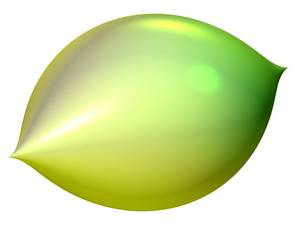# Description

In Algebra, one deals with the solutions of polynomial equations in one variable X, such as X2 - 2 = 0 or X5 + 4·X + 2 = 0. The set of all solutions of such polynomial equations with rational numbers as coefficients forms one field Qalg. This means that you can add, subtract, multiply, and divide the numbers in it. One of the main problems of Number Theory is to better understand the field Qalg. On the other hand, if polynomial equations (or systems of polynomial equations) are considered in several variables, their sets of solutions have a geometric structure (see the picture below). Also, e.g. the real solution set of X2 + Y2 - 1 = 0 is exactly the circle line. In addition, these sets of solutions also have a rich algebraic structure that results from considering polynomial equations. The branch of mathematics that investigates these sets of solutions is called Algebraic Geometry. A more detailed description can be found here."Zitrus" by Herwig Hauser is the real solution set of X2 + Z2 + Y3·(Y−1)3 = 0.

(Own work by User: Andreasmatt. Licensed under Creative Commons Attribution-Share Alike 3.0 via Wikimedia Commons https://commons.wikimedia.org/wiki/File:IMAGINARY_Zitrus_Herwig_Hauser.jpg)

Other algebraic areas that are the focus in Münster are Representation Theory, Group Theory and the Theory of Automorphic Forms. Of course, algebra is not an isolated individual science, but is closely related to other areas of mathematics (such as differential geometry, topology, logic, and operator algebras).

# Prerequisites

A lecture "Introduction to Algebra" with the following contents:

Groups, isomorphism theorems, abelian groups, permutation groups, group operations, and Sylow theorems. Rings, ideals, polynomial rings, Euclidean rings, principal ideal rings, factorial rings, divisibility in rings. Fields, field extensions and splitting fields. Galois extensions, Galois theory and applications.

This page presents the plan at the time of writing for the courses in future semesters. Please note that this plan is subject to change, and courses may be dropped, added, or modified in reaction to currently unforeseen events.

# We offer two specialisation modules:

• Specialisation in Group Theory and Representation Theory (GR)
• Specialisation in Number Theory and Arithmetic Geometry (AG)

### Courses for the specialisations in Group Theory and Representation Theory (GR) and in Number Theory and Arithmetic Geometry (AG)

Summer semester 2021

Prof. Dr. Christopher Deninger: Algebraic Number Theory II (Type I, AG, GR)
Prof. Dr. Peter Schneider: Galois representations and (phi, Gamma)-modules (Type I, II, AG, GR)

Winter semester 2021/2022

Dr. Robin Bartlett: Deformation theory of Galois representations (Type  II, AG, GR)
Prof. Dr. Linus Kramer: Geometric Group Theory I, possibly in German (Type I, II, GR)
Prof. Dr. Thomas Nikolaus: Algebraic Geometry (Type I, II, AG, GR)
PD Dr. Jakob Scholbach: Category Theory (Type I, II, AG, GR)
Prof. Dr. Dr. Katrin Tent: Geometric Group Theory: Hyperbolic groups and small cancellation (Type I, II GR)

Summer semester 2022

Prof. Dr. Thomas Nikolaus: Algebraic Geometry II (Type I, II AG, GR)
Dr. Bakul Sathaye: Geometric Group Theory II (Type I, II, GR)
apl. Prof. Dr. Wend Werner: Lie Theory and Quantization (Type I, II, GR)

Winter semester 2022/2023

Dr. Martin Bays: Geometric Group Theory I (GR, Type I, II)
Prof. Dr. Thomas Nikolaus: Algebraic Geometry 3 (Type I, II)
Prof. Dr. Eva Viehmann: Commutative Algebra (Type I, II)

Summer semester 2023

PD Dr. Lutz Hille: Linear Algebraic Groups (Type I, II)
Prof. Dr. Thomas Nikolaus: Algebraic Geometry 4 (Type I, II)

Winter semester 2023/2024

Prof. Dr. Eva Viehmann: Algebraic Geometry 1 (Type I, II)
N.N.: Commutative Algebra (Type I)

Summer semester 2024

Prof. Dr. Eva Viehmann: Algebraic Geometry 2 (Type I, II)

### Seminars

Summer semester 2021

Prof. Dr. Christopher Deninger: Zeta- and L-functions in algebraic number theory

Winter semester 2021/2022

Prof. Dr. Christopher Deninger: Class field theory

Summer semester 2022

Prof. Dr. Christopher Deninger: Elliptic curves
Prof. Dr. Eva Viehmann: Linear Algebraic Groups

# Further information

The research groups with algebraic orientation present in Münster cover large parts of modern algebra and number theory. The focus of the working groups are in detail:

RG Algebra and Number Theory (Prof. Deninger): Algebraic geometry, algebraic number theory
RG Algebra and Number Theory (Prof. Hartl): Algebraic geometry, arithmetic of function fields
RG Algebra and Number Theory (Prof. Hellmann): Algebraic geometry, Galois representations, p-adic automorphic forms
RG Algebra and Number Theory (Prof. Hille): Representation theory, toric algebraic geometry
RG Geometry, Topology and Group Theory (Prof. Kramer): Geometric group theory, Lie groups, theory of buildings
RG Homotopy Theory and Higher Algebra (Prof. Nikolaus): Homotopy theory, higher algebra, algebraic K-theory, field theories
RG Algebra and Number Theory (Prof. Schneider): Representation theory, p-adic analysis, Langlands program
RG Model Theory and Algebra (Prof. Tent): Group theory, mathematical logic, model theory

On the pages of these research groups you will find further information about lectures, seminars, master theses etc.